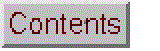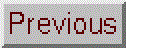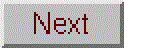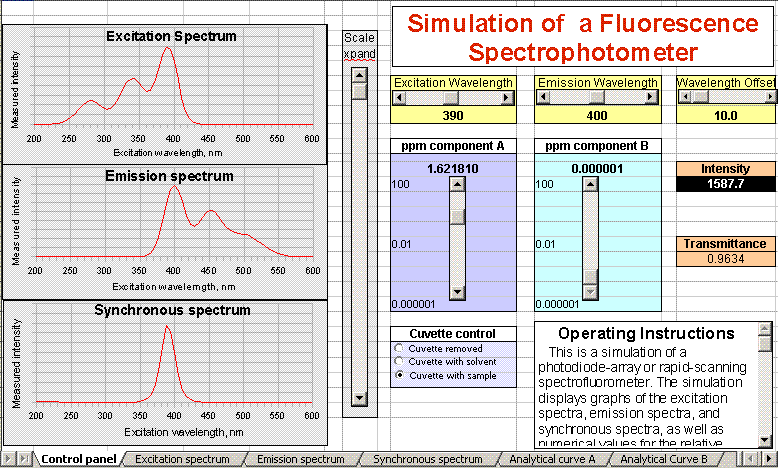##OpenOffice Version, July 2009.

Click to see a larger view.

Real-time simulation of a scanning fluorescence spectrofluorometer. Students can set the excitation and emission wavelengths, scan excitation spectra, emission spectra, or synchronous spectra, change the concentrations of two fluorescent components, insert and remove the blank and sample cuvettes, measure the wavelengths of maximum excitation and emission, Stokes shift, and detection limits, observe Raleigh and Raman scatter, dark current, photon noise, determine the frequency of the vibration causing the Raman peak, compare absorption to fluorescence measurement of the same solution, optimize measurement of two-component mixture by selective excitation and synchronous fluorescence methods, generate and plot analytical curves automatically, and observe the non-linearity and spectral distortion caused by self-absorption.

OpenOffice Version, FluorescenceOO.ods

WingZ Version 2.1: Fluor2.wkz
WingZ Version 3: FLUOR3.WKZ (with excitation-emission matrix contour plot);
WingZ player application and basic set of simulation modules, for windows PCs or Macintosh

Other related simulations:
Fluorescence Spectroscopy Signal-to-Noise Ratio (OpenOffice and Excel versions)
Multiwavelength Spectrometry (analysis of two-component mixture by absorption spectroscopy)

Operating Instructions, OpenOffice version:

This is a simulation of a photodiode-array or rapid-scanning spectrofluorometer. The simulation displays graphs of the excitation spectra, emission spectra, and synchronous spectra, as well as a numerical value for the relative fluorescence emission intensity (the black box labeled "Intensity").

To change the excitation and emission wavelengths (in nm), adjust the two sliders at the top, labeled "Excitation Wavelength" and "Emission Wavelength". To change the graph scale expansion, use the long vertical slider labeled "Scale xpand".  (This effects only the vertical intensity scale of the graphs, not the numerical intensity display).

To view these spectra enlarged, click the tabs at the bottom left of the window, labeled Control, Excitation, Emission, and Synchronous.

The sample solution may contain 1 or 2 fluorescent compounds, A and B, dissolved in water. You can control the concentrations of these two components by using the sliders labeled "ppm component A" and "ppm component B".  You can also control what is placed in the sample compartment with the "Cuvette control" buttons.

The transmittance of the sample solution at the excitation wavelength is also displayed, to aid in comparing fluorescence to absorption measurement and as an indication of the extend of the self-absorption or "inner filter" effect.

The synchronous fluorescence spectrum is displayed at the bottom left. The Wavelength Offset control (top right) controls the wavelength difference between the excitation and emission wavelengths in nm.

To inspect the analytical curve with respect to component A alone (at constant concentration of component B), click the tab at the bottom of the windows labeled "Analytical curve A".  To inspect the analytical curve with respect to component B alone (at constant concentration of component A), click the tab at the bottom of the windows labeled "Analytical curve B".

Operating instructions, WingZ version:

To change the concentrations of the components A and B, click on the up and down arrows to the left of the concentration displays(concentration range is zero to 100 ppm in a 1,2, 5, 10 sequence); or you can type in any arbitrary concentration for either component while the cuvette is removed.

Fluorescence intensity (in arbitrary units) and the absorbance of the solution at the excitation wavelength are displayed in the black boxes. Readings are continuous as long as the cuvette is inserted into the instrument. (The random fluctuations in readings are due to photon noise).

Clicking "Remove cuvette" simulates removal of the cuvette from the light path; the intensity read-out displays only the detector's dark current. Clicking "Insert blank" simulates inserting a cuvette filled with pure water into the light path; the intensity read-out displays the light scatter (Rayleigh and Raman) from the water. Clicking "Insert sample" simulates inserting a cuvette filled with a water solution of the two components at the specified concentrations. The cuvette must be removed to type in arbitrary concentrations and then inserted to measure.

To change the excitation and emission wavelengths (in nm), adjust the two sliders at the bottom. To scan a spectrum, click on the corresponding scan button. To obtain a synchronous spectrum, set the wavelength offset with the slider on the right and click "Scan both". Change the y-axis scale of the plots by clicking on one of the seven small "sensitivity" buttons labeled "10" through "3000", or press "auto" to allow the computer to automatically adjust the y-axis scale. Note: the intensity and absorbance displays respond immediately to changes in concentrations and wavelengths; however, spectra must be re-scanned after changing the concentrations, wavelengths, or offset.

Pressing "analyt.curve A" runs an analytical curve for component A and displays a log-log plot of intensity vs concentration of A from 0.001 to 100 ppm. Pressing "analyt. curve B" does the same thing for component B. Scanning a spectrum replaces the analytical curve plot. From this plot it is possible to convert the relative intensity readings into concentration in ppm.

Instructor's Notes:

This is a simulation of room temperature prompt fluorescence of two non-interacting fluorophors in aqueous solution with right angle geometry in a standard cuvette, measured with a corrected dispersive spectrofluorometer. You can think of the two components as two analytes or as one analyte (A) and a background or interfering component (B). Both components obey Vavilov's law (shapes of the emission spectra of each component separately are independent of the excitation wavelength, and vice versa, except for the scatter peaks). The simulation includes Rayleigh and Raman scatter peaks of the solvent (water); there is only one Raman band observable, that of the OH stretch of water. (The Rayleigh peak is fixed in amplitude but the Raman band height varies with the inverse 4th power of wavelength).  The Raman peak of water is often used to measure the sensitivity and signal-to-noise ratio of fluorescence instruments.

The simulation includes the self-absorption (inner-filter) effect for both the excitation beam and the fluorescence emission, and it includes photon noise but not flicker or detector (dark current) noise. In addition to an intensity display, there is also an absorbance readout, which gives the absorbance of the sample solution at the excitation wavelength; this is intended to allow a comparison of fluorescence to absorption measurement and as an indicator of the presence of self-absorption, but it is not a full simulation of absorption spectrophotometric measurement (it does not include stray light or finite spectra bandwidth deviations nor background shifts due to changes in cell transmission). The simulation has a synchronous scanning mode (constant delta-lambda). On most computers the scanning speed of the simulated instrument will be faster than typical real instruments.

There are several parameters that you can change, to modify the simulation experience for specific purposes. You can change the spectral characteristics of the two components. The excitation and the emission spectra are each modeled as three overlapping Gaussian bands. The heights, peak wavelengths, and widths of each band are given in the table at R20..R46. For example, h1ax is the height of the first band of component A's excitation spectrum, and w3bm is the width of the third band of component B's emission spectrum, and so forth (peak wavelengths and widths are in nm; height is in arbitrary units). You can change the overall signal-to-noise ratio of the instrument (cell Q17). You can also change the sequence of concentrations used to construct analytical curves (table in U10..U26 in the WingZ version and the large table starting at U8 in the OpenOffice version).

After making any changes, I suggest that you Save the simulation under a different file name, so you preserve the original.

Cell definitions and equations (for WingZ version 2.1):
```Inputs:

Concentration of A in ppm (cell I12)
Concentration of B in ppm (cell K12)
ex = wavelength of excitation monochromator (cell I8 or excitation slider)
em = wavelength of emission monochromator (cell K8 or emission slider)
of = synchronous offset (cell M8 or offset slider)
epsa = absorption coefficient of component A
epsb = absorption coefficient of component B
snr = signal-to-noise ratio (Cell Q17)
Z1 = 1 if cuvette is inserted; 0 if removed from the instrument.

Excitation band characteristics of component A:	(cells R20..R28)
band #     1     2       3
Height:   h1ax	h2ax	h3ax
Position: p1ax	p2ax	p3ax
Width:    w1ax	w2ax	w3ax

Emission band characteristics of component A: (cells R20..R28)
band #     1     2       3
Height:   h1am	h2am	h3am
Position: p1am	p2am	p3am
Width:    w1am	w2am	w3am

Excitation band characteristics of component B:	(cells R29..R37)
band #     1     2       3
Height:   h1bx	h2bx	h3bx
Position: p1bx	p2bx	p3bx
Width:    w1bx	w2bx	w3bx

Emission band characteristics of component B:	(cells R38..R46)
band #     1     2       3
Height:   h1bm	h2bm	h3bm
Position: p1bm	p2bm	p3bm
Width:    w1bm	w2bm	w3bm

U10..U26: sequence of component concentrations (ppm) for analytical curves.

Calculated quantities:

Concentration of A in ppb = A = 1000*ppmA
Concentration of B in ppb = B = 1000*ppmB
Wavelength of Raman peak in emission spectrum = raman = ex/(1-ex*0.00034)
Wavelength of Raman peak in excitation spectrum = xraman = em/(1-em*0.00034)
Intensity of Raman peak in emission spectrum = RamInt = 200000000000/ex^4
Intensity of Raman peak in excitation spectrum = xRamInt = 200000000000/em^4

Emission factor, component A
ema = (h1am*exp(-((em-p1am)/w1am)^2)
+h2am*exp(-((em-p2am)/w2am)^2)
+h3am*exp(-((em-p3am)/w3am)^2))

Emission factor, component B
emb = (h1bm*exp(-((em-p1bm)/w1bm)^2)
+h2bm*exp(-((em-p2bm)/w2bm)^2)
+h3bm*exp(-((em-p3bm)/w3bm)^2))

Excitation factor, component A
exa = (h1ax*exp(-((ex-p1ax)/w1ax)^2)
+h2ax*exp(-((ex-p2ax)/w2ax)^2)
+h3ax*exp(-((ex-p3ax)/w3ax)^2))

Excitation factor, component B
exb = (h1bx*exp(-((ex-p1bx)/w1bx)^2)
+h2bx*exp(-((ex-p2bx)/w2bx)^2)
+h3bx*exp(-((ex-p3bx)/w3bx)^2))

Absorbance of sample solution at the excitation wavelength
Aex = epsa*A*(h1ax*exp(-((ex-p1ax)/w1ax)^2)
+h2ax*exp(-((ex-p2ax)/w2ax)^2)
+h3ax*exp(-((ex-p3ax)/w2ax)^2))
+epsb*B*(h1bx*exp(-((ex-p1bx)/w1bx)^2)
+h2bx*exp(-((ex-p2bx)/w2bx)^2)
+h3bx*exp(-((ex-p3bx)/w3bx)^2))

Absorbance of sample solution at the emission wavelength
Aem = epsa*A*(h1ax*exp(-((em-p1ax)/w1ax)^2)
+h2ax*exp(-((em-p2ax)/w2ax)^2)
+h3ax*exp(-((em-p3ax)/w2ax)^2))
+epsb*B*(h1bx*exp(-((em-p1bx)/w1bx)^2)
+h2bx*exp(-((em-p2bx)/w2bx)^2)
+h3bx*exp(-((em-p3bx)/w3bx)^2))

Transmission of sample solution at the excitation wavelength
Tex = 10^(-Aex)

Transmission of sample solution at the emission wavelength
Tem = 10^(-Aem)

Total output intensity (fluorscence + scatter + Raman) (cell M13)
total = Z1*Tex*Tem*((A*ema*exa+B*emb*exb)
+100*exp(-((ex-em)/10)^2)
+RamInt*exp(-((em-raman)/10)^2))

Display outputs:

Absorbance (cell M20)
= Aex + 0.001*(rand()-0.5)

Intensity (cell M12)
=abs(total+(sqrt(total)+2)*(rand())/snr)

Array calculations:

D31..D101: wavelength, 200..600 nm in 6 nm steps

B31..B101: absorbance of solution at wavelength
absorbance = epsa*A*(h1ax*exp(-((wavelength-p1ax)/w1ax)^2)
+h2ax*exp(-((wavelength-p2ax)/w2ax)^2)
+h3ax*exp(-((wavelength-p3ax)/w3ax)^2))
+epsb*B*(h1bx*exp(-((wavelength-p1ax)/w1bx)^2)
+h2bx*exp(-((wavelength-p2ax)/w2bx)^2)
+h3bx*exp(-((wavelength-p3ax)/w3bx)^2))

C31..C101: transmission of solution at wavelength
transmission = 10^(absorbance)

E31..E101: excitation spectrum (including Rayleigh and Raman scatter)
excitation = Tem*transmission*(A*((h1ax*exp(-((wavelength-p1ax)/w1ax)^2)
+h2ax*exp(-((wavelength-p2ax)/w2ax)^2)
+h3ax*exp(-((wavelength-p3ax)/w3ax)^2))*ema)
+B*((h1bx*exp(-((wavelength-p1bx)/w1bx)^2)
+h2bx*exp(-((wavelength-p2bx)/w2bx)^2)
+h3bx*exp(-((wavelength-p3bx)/w3bx)^2))*emb)
+100*exp(-((wavelength-em)/10)^2)
+xRamInt*exp(-((wavelength-xraman)/10)^2))

G31..G101: excitation spectrum with photon noise
ex+noise = \$Z\$1*(abs(excitation+(sqrt(excitation)+2)*(rand())/snr))

I31..I101: emission spectrum (including Rayleigh and Raman scatter)
emission  = Tex*transmission*(A*(exa*(h1am*exp(-((wavelength-p1am)/w1am)^2)
+h2am*exp(-((wavelength-p2am)/w2am)^2)
+h3am*exp(-((wavelength-p3am)/w3am)^2)))
+B*(exb*(h1bm*exp(-((wavelength-p1bm)/w1bm)^2)
+h2bm*exp(-((wavelength-p2bm)/w2bm)^2)
+h3bm*exp(-((wavelength-p3bm)/w3bm)^2)))
+100*exp(-((wavelength-ex)/10)^2)
+RamInt*exp(-((wavelength-raman)/10)^2))

K31..K101: emission spectrum with photon noise
em+noise = \$Z\$1*(abs(emission+(sqrt(emission)+2)*(rand())/snr))

Transmission at offset wavelength (wavelength+offset)
A31..A101: Toff
Toff = 10^(-epsa*A*(h1ax*exp(-((wavelength-p1ax+of)/w1ax)^2)
+h2ax*exp(-((wavelength-p2ax+of)/w2ax)^2)
+h3ax*exp(-((wavelength-p3ax+of)/w3ax)^2))
+epsb*B*(h1bx*exp(-((wavelength-p1ax+of)/w1bx)^2)
+h2bx*exp(-((wavelength-p2ax+of)/w2bx)^2)
+h3bx*exp(-((wavelength-p3ax+of)/w3bx)^2)))

M31..M101: synchronous spectrum (including Rayleigh and Raman scatter)
synch = Toff*transmission*(A*((h1ax*exp(-((wavelength-p1ax)/w1ax)^2)
+h2ax*exp(-((wavelength-p2ax)/w2ax)^2)
+h3ax*exp(-((wavelength-p3ax)/w3ax)^2))
*(h1am*exp(-((wavelength-p1am+of)/w1am)^2)
+h2am*exp(-((wavelength-p2am+of)/w2am)^2)
+h3am*exp(-((wavelength-p3am+of)/w3am)^2)))
+B*((h1bx*exp(-((wavelength-p1bx)/w1bx)^2)
+h2bx*exp(-((wavelength-p2bx)/w2bx)^2)
+h3bx*exp(-((wavelength-p3bx)/w3bx)^2))
*(h1bm*exp(-((wavelength-p1bm+of)/w1bm)^2)
+h2bm*exp(-((wavelength-p2bm+of)/w2bm)^2)
+h3bm*exp(-((wavelength-p3bm+of)/w3bm)^2)))
+100*exp(-((of)/10)^2)
+RamInt*exp(-((wavelength+of-(wavelength/(1-wavelength*0.00034)))/10)^2))

O31..O101: synchronous spectrum with photon noise
synch+noise = \$Z\$1*(abs(synch+(sqrt(synch)+2)*(rand()/snr)))

Graphs:

Excitation spectrum: excitation+noise vs excitation wavelength
Emission spectrum: emission+noise vs emission wavelength
Synchronous spectrum: sync+noise vs excitation wavelength
Analytical curves: Intensity vs concentration of A or B in ppm
```

`Student assignment, WingZ version:`
Simulation of Scanning Fluorescence Spectrometer

This is a simulation of a scanning spectrofluorometer.  The simulation displays excitation spectra, emission spectra, and synchronous spectra, relative fluorescence intensity, and absorbance at the excitation wavelength. Operating instructions are contained in the scrolling text field in the upper right of the screen.  Answer the following questions on a separate sheet to turn in.  Please do not make  repeated print-outs of this spreadsheet.

1. Set A=1 ppm and B=0. Determine the wavelengths of maximum excitation and emission for component A. What is its Stokes shift?

2. Does Vavilov's Law* seem to hold for compound A, that is, is the shape of the emission spectra independent of the excitation wavelength, and vice versa, except for the scatter peaks?

3. Is there any sign of Rayleigh or Raman scatter? How could you distinguish these from genuine fluorescence?

4. Check the blank (click on "Insert Blank"). Increase the sensitivity setting as necessary. Is there any sign of dark current or background fluorescence? What are the main features of the excitation and emission spectra of the blank. Estimate the spectral bandpass of the monochromators.

5. Does the wavelength separation between the Rayleigh and Raman scatter peaks in the emission spectrum vary with excitation wavelength? What is the frequency, in cm-1, of the vibration causing the Raman peak? What vibration is most likely the cause?

6. Find the combination of excitation and emission wavelength that gives the best precision of measurement of low concentrations of component A. Estimate the detection limit of component A in ppm. Is the detection limit lower by fluorescence or by absorption measurement? By approximately what factor?

7. Over most of the concentration range, what is the source of noise in the intensity readings and in the spectra? How could you prove this?

8. Is there evidence of non-linearity in the relationship between concentration and intensity at high concentrations? What is the most likely source of the non-linearity?

9. Vary the wavelength offset and observe the synchronous spectrum. What offset gives the largest peak height? Explain the effect of Rayleigh and Raman scatter on the synchronous spectrum. Note: this is a constant wavelength synchronous spectrum.

10. Set A=0 and B=1 ppm. Determine the wavelengths of maximum excitation and emission for component B. What is its Stokes shift? Can mixtures of these two components be determined by fluorescence measurement?

* Vavilov's Law states that the shape of the fluorescence emission spectrum of a single fluorophor is independent of the excitation wavelength, and vice versa.  This holds only for the fluorescence peaks, not Raman or scatter peaks.

1. Question: Why is fluorescence intensity measured in "arbitrary units"?
Answer: Nothing more is needed for the common applications of fluorescence spectroscopy: for quantitative applications, a calibration curve can be prepared and used on the same instrument employing any set of intensity units equally well, and for qualitative applications, the shape of a spectrum is the same no matter what intensity units are used. Calibrating a fluorescence spectrophotometer to read in absolute physical units (watts, for example) would add to the cost and would not result in any real advantage for most applications. Most fluorescence spectrophotometers simply measure the detector signal, which is proportional to the fluorescence intensity. The magnitude of that signal at a given analyte concentration is somewhat arbitrary, depending upon the choice of detector and its operating conditions, as well as the intensity of the light source and the characteristics of the  monochromator and the rest of the optics. See "Signal-to-Noise Ratio and Detection Limit of Fluorescence Spectroscopy" for a detailed analysis of the factors influencing the detector signal.

2. Question: Why not measure absorbance or transmission, as in absorption spectroscopy?
Answer: This is due to the different optical configuration of absorption and fluorescence measurement. In absorption spectrometry, the light beam that passes through the sample is measured directly by a detector placed a 180º angle from the incident beam, which allows the incident beam intensity to be measured simply by replacing the sample with a "blank".  In fluorescence spectrometry, the light beam that passes through the sample is not measured at all; rather, the fluorescence emission is measured, usually at a 90º angle from the incident beam. Therefore, absorbance or transmission can not be measured in a fluorescence spectrometer because the detector does not measure the incident beam. Even if you tried to move the detector around to 180º to measure the incident beam, it wouldn't work very well because the light intensity of the incident beam can be as much as 1013 times brighter than the fluorescence intensity, and no detector in common use can measure intensities over that wide a range.  (Note: some instruments do sample a small portion of the incident bean and measure it with a second (lower sensitivity) detector, then calculate the ratio of the fluorescence intensity to the incident intensity.  Even this ratio, however, is not comparable between instruments, in the same way that absorbances can be compared

3. Question: How does fluorescence intensity vary with concentration?
Answer:  Fluorescence intensity is directly proportional to concentration (i.e. the calibration curve is linear), as long as the absorbance of the analyte at the excitation wavelengths is very low, say, less than about 0.004 (99% T).  If the absorbance is greater than this, then the excitation intensity is reduced by the absorption, and the calibration curve becomes non-linear (concave down). The effect is exacerbated if the analyte also absorbs at the emission wavelength.

4. Question: Why is fluorescence measured at a 90º angle to the incident (excitation) beam?
Answer:  There is nothing magic about 90º.  The fluorescence can't be measured at a 180º, because the transmitted beam would completely swamp the fluorescence. The choice of  90º is a matter of convenience, especially when conventional square cross-section cuvettes are used.

5. Question: What are the relative detection limits of absorption and fluorescence measurement?
Answer:  This depends largely on the fluorescence quantum efficiency of the analyte (the ratio of the number of photons emitted as fluorescence to the number absorbed). For a highly fluorescent molecule such as quinine, which has a quantum efficiency of 0.5, fluorescence has a better (lower) theoretical detection limit than absorption by a factor of roughly 103 using comparable instrumentation, as you can demonstrate by comparing  AbsorptionSNR.ods to FluorescenceSNR.ods. But most fluorescent molecules have quantum efficiencies lower than 0.5, so the advantage of fluorescence is not so large on average. But by the same token, any molecule with a quantum efficiency higher than 0.0005 is likely to be at least marginally better by fluorescence, at least in terms of theoretical detection limit.

6. Question: How can a fluorescent peak can be distinguish from an Raman peak?
Answer: Vary the excitation wavelength slightly. If the peak shifts in wavelength and its wavelength is longer than the excitation wavelength, it's a Raman peak; if it changes its intensity but not its wavelength, it's a fluorescent peak.

7. Question: Why choose a fluorescence measurement over absorption?  After all, any molecule that fluoresces must also absorb.
Answer: The choice depends on the fluorescence characteristics of the analyte and the matrix that it is present in. If the analyte exhibits fluorescence at excitation and emission wavelengths within the range of your instrument, then it is a candidate for fluorescence measurement, if its quantum efficiency is high enough. But a further requirement is that the fluorescence emissions of other chemical components in the sample not interfere, either because they are very weak or because the excitation or emission wavelengths of those components can be successfully resolved from that of the analyte.  Each case has to be evaluated individually. Usually a fluorescence measurement is chosen over an absorption measurement when the analyte is strongly fluorescent and the other components in the sample have absorption that interfere with an absorption measurement but do not exhibit strong fluorescence emission that would interfere with a fluorescence measurement.
(c) 1991, 2015. This page is part of Interactive Computer Models for Analytical Chemistry Instruction, created and maintained by Prof. Tom O'Haver , Professor Emeritus, The University of Maryland at College Park. Comments, suggestions and questions should be directed to Prof. O'Haver at toh@umd.edu. Number of unique visits since May 17, 2008: